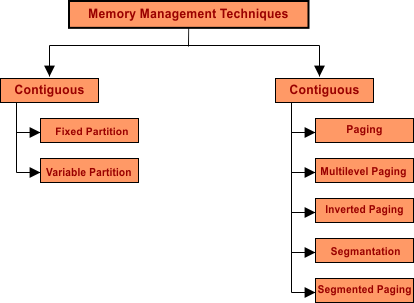Select Page

# Memory Management And Its Techniques

Memory management is the basic concept of  OS which  manages the main memory.  Allocation and de-allocation of process takes place in-between main memory and secondary memory. Memory management keeps the record of each process in main memory.

In this lecture, We will cover the  basic concepts of Memory Management. Our basic goal is to load more and more processes in main memory which is also called degree of multi-programming. First look at the concept of  degree of multi-programming.

## What Is The Degree Of Multi-Programming?

The degree of multi-programming describes the maximum number of processes that a single-processor system can accommodate efficiently.

In simple words, Load more and more programs from secondary memory to main memory. So that the efficiency of system is increase. This is multi-programming or degree of multi-programming.

Goal: Memory utilization maximum

The basic Purpose of degree of multi-programming, is the Maximum utilization of CPU.

### How to Improve Degree Of Multi-Programming?

Answer is by increasing size of RAM.

Explain with Example:

As we know, every process is executed by CPU and I/O.

If K is the amount of time in which Process/program is executed by I/O event/operation then,

CPU utilization Time = 1- K (1 is the unit time to complete entire process)

If there are different Number of processes in the RAM then CPU utilization time is given below in the table. Suppose I/O utilization is 70% (K=70%) of each process then CPU utilization is

 Processes CPU utilization Time If only 1 process in RAM then CPU utilization will be 1-K1 = 1-(0.70)1 = 0.30 = 30% If 2 processes in RAM then CPU utilization will be 1-K2= 1-(0.70)2 = 76% If 5 processes in RAM then CPU utilization will be 1-K5= 1-(0.70)5 = 83% If 10 processes in RAM then CPU utilization will be 1-K10= 1-(0.70)10= 96%

Note: No of process=10, means that there are 10 processes in main memory.

Conclusion: Increase the size of RAM so that more and more process can accommodate in main memory then utilization of CPU becomes maximum.

So, higher the degree of multi-programming higher will be the CPU utilization.

### Memory Management Technique1. Contiguous

i. Fixed memory Partitions
ii. Variable memory Partitions

2. Non Contiguous

i. Paging
ii. Multilevel Paging
iii. Inverted paging
iv. Segmentation
v. Segmented Paging

In upcoming lectures, we will see one by one all non-contiguous

Help Other’s By Sharing…